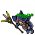## Wednesday, January 13, 2021

### TibiaGoals Riddles E5R2

Comment down below!

1.Lovely Olka

2.8.544
Holistico

3.5!
Holistico
(The other answer was my dog)

4.Purple line = 5

Shizurie

5.Character - Ragge Storm

I think the answer is 4 (purple line)

1.I'm sorry, the correct answer is 5. However you still have chances to take part in the lottery! See you on Sunday!

6.Purple line = 5 (dark Bombox) 💜

7.Purple line = 5 (dark Bombox) 💜

8.The purple line's length is 5. :) Thanks for hosting the riddle!

9.Nehkz
Relania

3^2+4^2=5^2

The purple line is 5.

10.Nehkz
Relania

3^2+4^2=5^2

The purple line is 5.

11.Nehkz
Relania

3^2+4^2=5^2

The purple line is 5.

12.Nehkz
Relania

3^2+4^2=5^2

The purple line is 5.

13.14.15.1.Sorry to say, your answer wasn't correct. However you still have chance to take part in lottery! See you on Sunday!

16.17.Char: Ghak Besahalia (Talera)

18.19.Skyelie

20.5 - Chapa Drunk

21.5 - Chapa Drunk

22.5 - Chapa Drunk

23.Sir Pitillox

5

24.25.5 Kidler Zaklinacz

26.27.5 - Myrkz (Wintera)

28.Aribebech : 5

29.30.31.Purple line = 5

32.33.1.34.5
Character name: Kutarate Fighter

35.5 - Knight Narco

36.37.5
Sir wuachachucho

38.39.40.41.1.42.Purple line = 5

Character: Elee Bae

43.Assuming the blue line cuts the pink line in the middle of it, the length of the purple line is 5.

-Essiee

44.Char: Dyaniixz

45.R= 5
Samuray de carro

46.You can resolve this by the Pythagorean theorem:

c^2 = a^2 + b^2
c = hypotenuse (the side we want to know)

a and b = the other sides.

So we have:
a = 4
b = 3

c^2 = 4^2 + 3^2
c = 5

purple line = 5.

Character: Amy Meow

47.You can resolve this by the Pythagorean theorem:

c^2 = a^2 + b^2
c = hypotenuse (the side we want to know)

a and b = the other sides.

So we have:
a = 4
b = 3

c^2 = 4^2 + 3^2
c = 5

purple line = 5.

Character: Amy Meow

48.Nick:Nikito The Wise

49.Nick: Nikito The Wise

50.Character:Paollah

51.1.52.The answer is 5 * (if I saw right I missclicked & wrote the wrong number on my first comment)

Char: Dyaniixz

53.5 Bombillito

54.Purple Line = 5 .......zazy torturador sombrio

55.Respuesta es 5 .... Bebitha consentida

56.